Hostname: page-component-797576ffbb-jhnrh Total loading time: 0 Render date: 2023-12-07T23:32:02.194Z Has data issue: false Feature Flags: { "corePageComponentGetUserInfoFromSharedSession": true, "coreDisableEcommerce": false, "useRatesEcommerce": true } hasContentIssue false

# Bounds for Euler from vorticity moments and line divergence

Published online by Cambridge University Press:  24 July 2013

## Abstract

The inviscid growth of a range of vorticity moments is compared using Euler calculations of anti-parallel vortices with a new initial condition. The primary goal is to understand the role of nonlinearity in the generation of a new hierarchy of rescaled vorticity moments in Navier–Stokes calculations where the rescaled moments obey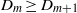${D}_{m} \geq {D}_{m+ 1}$, the reverse of the usual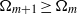${\Omega }_{m+ 1} \geq {\Omega }_{m}$ Hölder ordering of the original moments. Two temporal phases have been identified for the Euler calculations. In the first phase the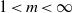$1\lt m\lt \infty$ vorticity moments are ordered in a manner consistent with the new Navier–Stokes hierarchy and grow in a manner that skirts the lower edge of possible singular growth with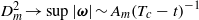${ D}_{m}^{2} \rightarrow \sup \vert \boldsymbol{\omega} \vert \sim A_{m}{({T}_{c} - t)}^{- 1}$ where the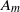${A}_{m}$ are nearly independent of$m$. In the second phase, the new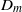${D}_{m}$ ordering breaks down as the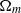${\Omega }_{m}$ converge towards the same super-exponential growth for all$m$. The transition is identified using new inequalities for the upper bounds for the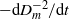$- \mathrm{d} { D}_{m}^{- 2} / \mathrm{d} t$ that are based solely upon the ratios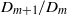${D}_{m+ 1} / {D}_{m}$, and the convergent super-exponential growth is shown by plotting$\log (\mathrm{d} \log {\Omega }_{m} / \mathrm{d} t)$. Three-dimensional graphics show significant divergence of the vortex lines during the second phase, which could be what inhibits the initial power-law growth.

## JFM classification

Type
Rapids
Information
Journal of Fluid Mechanics , 25 August 2013 , R2

## Access options

Get access to the full version of this content by using one of the access options below. (Log in options will check for institutional or personal access. Content may require purchase if you do not have access.)

## References

Beale, J. T, Kato, T. & Majda, A. 1984 Remarks on the breakdown of smooth solutions of the 3-D Euler equations. Commun. Math. Phys. 94, 6166.Google Scholar
Bustamante, M. D. & Kerr, R. M. 2008 3D Euler about a 2D symmetry plane. Physica D 237, 19121920.Google Scholar
Deng, J., Hou, T. Y. & Yu, X. 2005 Geometric properties and nonblowup of 3D incompressible Euler flow. Comm. Part. Diff. Equ. 30, 225243.Google Scholar
Doering, C. R. 2009 The 3D Navier–Stokes problem. Annu. Rev. Fluid. Mech. 41, 109128.Google Scholar
Donzis, D., Gibbon, J. D., Kerr, R. M., Pandit, R., Gupta, A. & Vincenzi, D. 2013 Rescaled vorticity moments in the 3D Navier–Stokes equations. J. Fluid Mech. (submitted), arXiv:1302.1768.Google Scholar
Gibbon, J. D. 2012 Conditional regularity of solutions of the three-dimensional Navier–Stokes equations and implications for intermittency. J. Math. Phys. 53, 115608.Google Scholar
Gibbon, J. D. 2013 Dynamics of scaled norms of vorticity for the three-dimensional Navier–Stokes and Euler equations. Procedia IUTAM 7, 4958.Google Scholar
Hou, T. Y. 2008 Blow-up or no blow-up? The interplay between theory and numerics. Physica D 237, 19371944.Google Scholar
Kerr, R. M. 1993 Evidence for a singularity of the three-dimensional, incompressible Euler equations. Phys. Fluids A 5, 17251746.Google Scholar
Kerr, R. M. 1996 Cover illustration: vortex structure of Euler collapse. Nonlinearity 9, 271272.Google Scholar
Kerr, R. M. 2011 Vortex stretching as a mechanism for quantum kinetic energy decay. Phys. Rev. Lett. 106, 224501.Google Scholar
Kerr, R. M. 2012 Dissipation and enstrophy statistics in turbulence: are the simulations and mathematics converging? J. Fluid Mech. 700, 14.Google Scholar
Kerr, R. M. 2013 Swirling, turbulent vortex rings formed from a chain reaction of reconnection events. Phys. Fluids 25, 065101.Google Scholar
Yeung, P. K., Donzis, D. A. & Sreenivasan, K. R. 2012 Dissipation, enstrophy and pressure statistics in turbulence simulations at high Reynolds numbers. J. Fluid Mech. 700, 515.Google Scholar